# Sum Values by Group

This post will guide you how to sum data by group in a table in Excel. Assuming that you have a table that contain the product name and its sales result. And you have group by those values based on the product name, then you want to sum values based one each product name. You can create a new Excel formula based on the IF function and SUMIF function.

Here is a table that contain product name and sales:

 Name Sales Excel \$45.00 Excel \$34.00 Excel \$66.00 Word \$435.00 Word \$443.00 Word \$23.00

## Sum Values by Group

The data of the above table have been grouped by the product name. So you can write down the following formula using the IF function and SUMIF function. Then type this formula into the formula box in Cell D2.

`=IF(B2=B1," ",SUMIF(B:B,B2,C:C))`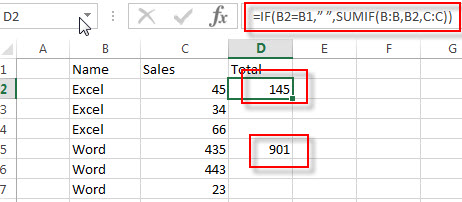Let’s see how this formula works:

=SUMIF(B:B,B2,C:C)

This function will sum cell values that meet a single criteria. It will check each value in column B to see if its value is equal to the value of Cell B2. If TRUE, then sum its sales value in corresponding Cell in column C.  And this function will be called by IF function.

The IF function will test each value in column B to check if its value is same as the value in the above cell. For example, if the value of Cell B2 is equal to Cell B1, then the SUMIF function will be run. And the total values of the same product will be returned.

## Sum Values by group with Subtotal feature

You can also use the subtotal feature to achieve the same result to sum the values by group. Just do the following steps:

#1 go to DATA tab in Excel’s ribbon toolbar.

#2 select the range of cells that contain product names (B2:B7)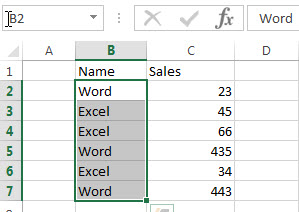#3 click Sort command under Sort&Filter group.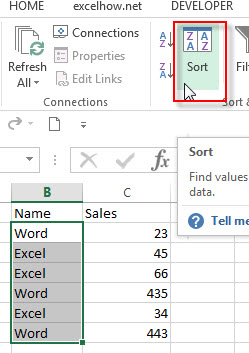#4 sort by the product name column. Click OK button.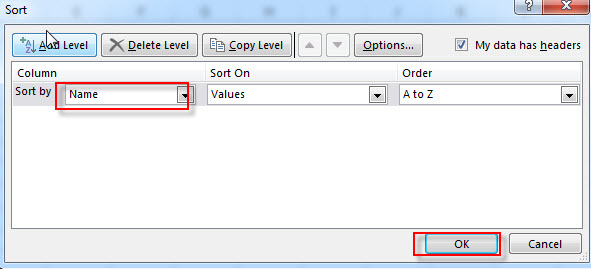#5 the data has been grouped by the product name.

#6 select all values including product name and its sales value.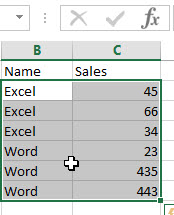#7 click Subtotal command under Outline group. Click OK.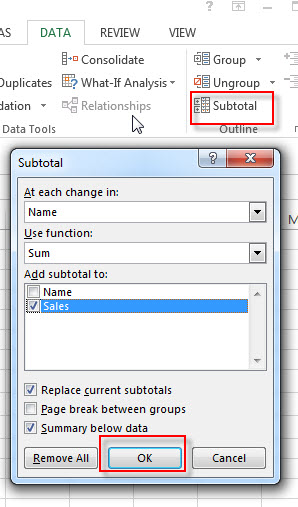#8 the total values of each product name has been summed.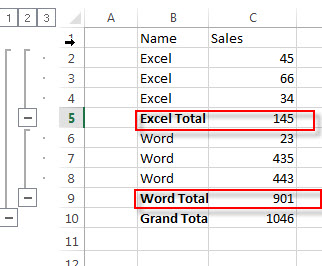### Related Functions

• Excel SUMIFS Function
The Excel SUMIFS function sum the numbers in the range of cells that meet a single or multiple criteria that you specify. The syntax of the SUMIFS function is as below:=SUMIFS (sum_range, criteria_range1, criteria1, [criteria_range2, criteria2], …)…
• Excel SUMIF Function
The Excel SUMIF function sum the numbers in the range of cells that meet a single criteria that you specify. The syntax of the SUMIF function is as below:=SUMIF (range, criteria, [sum_range])…
• Excel IF function
The Excel IF function perform a logical test to return one value if the condition is TRUE and return another value if the condition is FALSE. The IF function is a build-in function in Microsoft Excel and it is categorized as a Logical Function.The syntax of the IF function is as below:= IF (condition, [true_value], [false_value])….